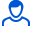Content: teorver_5588.doc (28.50 KB)

Positive responses: 0
Negative responses: 0

Refunds: 05588. x̅=10, n=49, σ=7. Find the confidence interval for estimating with a reliability of 0.9 the unknown mathematical expectation a of a normally distributed feature X of the general population, if the general standard deviation σ=7, the sample mean x̅v=10 and the sample size n=49 are known.
Detailed solution. Decorated in Microsoft Word 2003 (Quest decided to use the formula editor)
No feedback yet